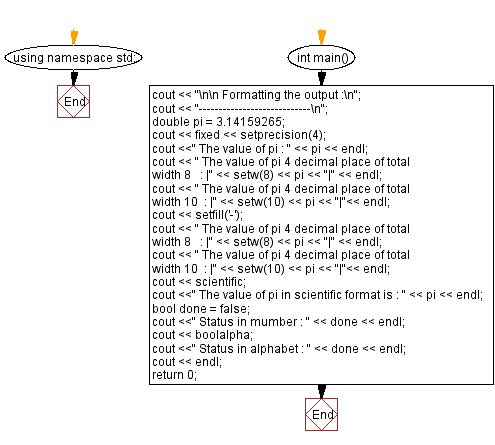﻿ C++ Exercises: Formatting the output - w3resource

# C++ Exercises: Formatting the output

## C++ Basic: Exercise-10 with Solution

Write a C++ program to format the output.

Sample Solution:

C++ Code :

``````#include <iostream>
#include <iomanip>    // Needed to do formatted I/O
using namespace std;

int main()
{
cout << "\n\n Formatting the output :\n";
cout << "----------------------------\n";

double pi = 3.14159265; // this is floating point number
cout << fixed << setprecision(4); // number is set to display with 4 decimal places
cout <<" The value of pi : " << pi << endl;
cout << " The value of pi 4 decimal place of total width 8   : |" << setw(8) << pi << "|" << endl; // setw() sets the total width
cout << " The value of pi 4 decimal place of total width 10  : |" << setw(10) << pi << "|"<< endl;

cout << setfill('-'); // setfill() sets to fill the blanks with specified character
cout << " The value of pi 4 decimal place of total width 8   : |" << setw(8) << pi << "|" << endl;
cout << " The value of pi 4 decimal place of total width 10  : |" << setw(10) << pi << "|"<< endl;

cout << scientific;  // set value in scientific format with exponent
cout <<" The value of pi in scientific format is : " << pi << endl;

bool done = false; // this is boolean variable
cout <<" Status in number : " << done << endl;
cout << boolalpha;     // set output in alphabet true or false
cout <<" Status in alphabet : " << done << endl;
cout << endl;
return 0;
}
``````

Sample Output:

``` Formatting the output :
----------------------------
The value of pi : 3.1416
The value of pi 4 decimal place of total width 8   : |  3.1416|
The value of pi 4 decimal place of total width 10  : |    3.1416|
The value of pi 4 decimal place of total width 8   : |--3.1416|
The value of pi 4 decimal place of total width 10  : |----3.1416|
The value of pi in scientific format is : 3.1416e+00
Status in mumber : 0
Status in alphabet : false
```

Flowchart:C++ Code Editor:

What is the difficulty level of this exercise?

﻿

## C++ Programming: Tips of the Day

Why is there no std::stou?

The most pat answer would be that the C library has no corresponding "strtou", and the C++11 string functions are all just thinly veiled wrappers around the C library functions: The std::sto* functions mirror strto*, and the std::to_string functions use sprintf.

Ref: https://bit.ly/3wtz2qA

We are closing our Disqus commenting system for some maintenanace issues. You may write to us at reach[at]yahoo[dot]com or visit us at Facebook